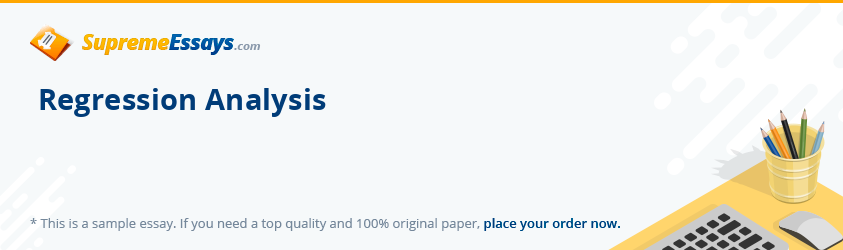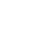Live chatIn the field of statistics regression analysis uses the techniques of modeling and analyzing multiple variables focusing on the relationships between dependent and independent variables helping the analyst to understand how the change of criterion in one independent variable affects the criterion of other dependent variables. In the process it determines the average values of dependent variables. The target is regression function and probability distribution. Used widely for prediction and forecasting, regression analysis is also used for exploring relationships. Several techniques have been evolved including linear regression, ordinary least square regression, and nonparametric regression. According to Lane D, when two variables are related, prediction of any person's score on one of the variables from the score on the second variable has a good chance of being accurate. Assumption adopted by Lane was that the relationship between the two variables was linear in nature. "Given that the relationship is linear, the prediction problem becomes one of finding the straight line that best fits the data. Since the terms "regression" and "prediction" are synonymous, this line is called the regression line". Methods of simple regression and linear regressions were clearly explained in the works of Waner S who also brought up the Regression Calculator.Regression AnalysisIn the field of statistics regression analysis refers to the techniques for modeling as well as analyzing multiple variables. Focus of the analysis is always on the relationships that exist between dependent and independent variables. Such analysis helps the researcher to clearly apprehend the ways of changes in values of the dependent variables when values of one or more dependent variables fluctuate.For instance, the methodology used in the analysis of heart study data is "a standard analysis of the Framingham Heart Study data is a generalized person-years approach in which risk factors or covariates are measured every two years with a follow-up between these measurement times to observe the occurrence of events such as cardiovascular diseases." (Source: RB D'Agostino, M Lee, AJ Belanger, LA Cupples, Statistics - How Many Subjects Do it Take to Do a Regression Analysis" - 1990)According to Lane D, when two variables are related, prediction of any person's score on one of the variables from the score on the second variable has a good chance of being accurate. Assumption adopted by Lane was that the relationship between the two variables was linear in nature. "Given that the relationship is linear, the prediction problem becomes one of finding the straight line that best fits the data. Since the terms "regression" and "prediction" are synonymous, this line is called the regression line".In explaining the mathematical representation of the regression line predicting Y from X is Y'=bX + A; where X is the variable represented on the abscissa (X-axis), b is the slope of the line, A is the Y intercept and Y' consists of the predicted values of Y for the various values of X.As illustration, Lane advances the following example where the relationships between identical blocks tests measuring spatial ability and Wonderlic test measuring general intelligence is analyzed and represented. It seems that the relationship is fairly strong in the present case at 0.677. In the process he also displays the best fitting straight line with a slope of 0.481 and Y intercept of 15.8468 and the regression line can be used for predicting. Scores in the graph for Wonderlic is 10 while on Identical Block is 20.From the formula for Y', it can be calculated that the predicted score is 0.481 x 10 + 15.86 = 20.67. The conclusion derived by Lane is as follows: -

Limited time Offer

0
0
days
:
0
0
hours
:
0
0
minutes
:
0
0
seconds
Get 19% OFF

"When scores are standardized, the regression slope (b) is equal to Pearson's r and the Y intercept is 0 . This means that the regression equation for standardized variables is:Y' = rX." Besides use in prediction, the regression line can also usefully describe relationship between two variables with the slope revealing the change in criterion of unit and its effect on the predictor variable.Method of simple regression and linear regression has been clearly explained by Waner S in his "Regression Calculator"."Enter your values for x and y below (leave the third column blank -- this will show the values predicted by the regression model). Arithmetic expressions such as 2/3 or 3+(4*pi) are fine. Then press the button (in the top right-hand frame) corresponding to the kind of regression equation you want. (For example, press the " y = mx + b" button for linear regression.) After that, you can obtain a graph of the points you entered and the regression curve by pressing "Graph."In the examples provided by Waner, the algorithm is written so that it would round the output to not more than eleven significant digits. To provide the best fit line or the regression line he comes up with the example of price in comparison to sales of new homes during a particular year as the following table indicates.Price (Thousands of \$)Sales of New Homes This YearSimplifying the situation by replacing each of the price ranges by only one that is present in the middle of the range, the following table is derived:Price (Thousands of \$)Sales of New Homes This YearOne can use these data to construct a demand function for the real estate market where the demand is Y and sales is represented by X. "The data definitely suggest a straight line, more-or-less, and hence a linear relationship between p and q. Here are several possible "straight line fits." (Wane S - 2007)The question that invariably arises is which one is the best fitted line or regression line in the above graph. Sales can be predicted by best-fit-line or the predicted value and it should be as close as possible to actual or observed values. Differences between the two appear in the following graph as vertical lines:Objective of analyzer is to make the vertical distance as small as possible though they cannot be set to zero. In such case a straight line would have passed through the data points. That is not the case here. So the only possible alternative is finding out the line that minimizes the distances. However all the distances cannot be minimized and therefore the solution is minimizing some reasonable combination of them such as their sum. Once again that would be difficult since distances are measure in terms of absolute values. Hence the adopted method should be adopting the sum of the squares of the distances without any absolute value."The line that minimizes this sum is called the best fit line, regression line, or least squares line associated with the given data."

0

Preparing Orders

0

Active Writers

0%

Positive Feedback

0

Support Agents

Limited offerget 15% off your 1st order with code first15